## Exploratory Data Analysis & VisualizationTelco

# Using Data Analytic Techniques To Visualize Telco Churn

The datasets and complete code can be found at the link below.

The method I am using to analyze the dataset is by using Data Science OSEMN framework. It can be best explained by this article writen by Dr. Lau here.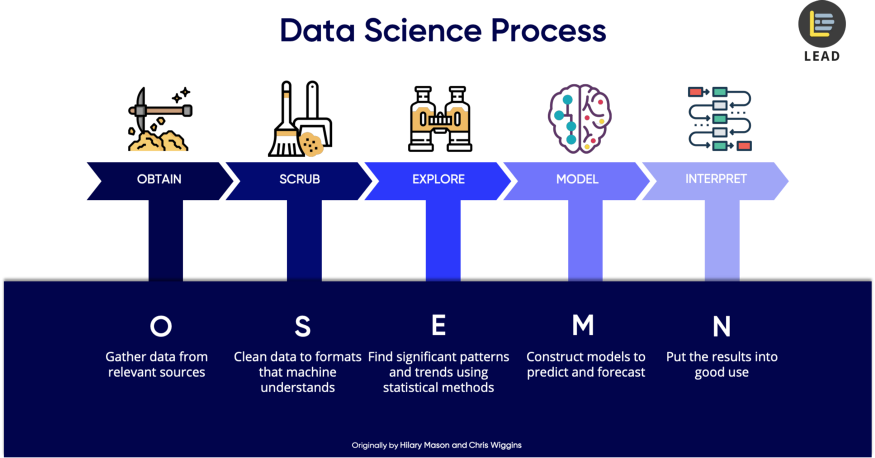Importing Python Libraries
First, we need to import the necessary python libraries for this analysis. These include the basic packages such pandas, seaborn and matplotlib.

import pandas as pd
import seaborn as sns
import matplotlib.pyplot as plt

Data Source
The data that I am using for this portfolio is the raw dataset sample given in the class as per link dataset before. In a quick glance it contains columns and rows with mostly words & numbers in the table. I will use Python to perform all data analysis and visualization. This data is originally in a CSV file and we will convert it into a data frame by using Pandas.

master/telco_customer_churn_dataset.csv')

Let’s take a look at what we have.

df.sample(5)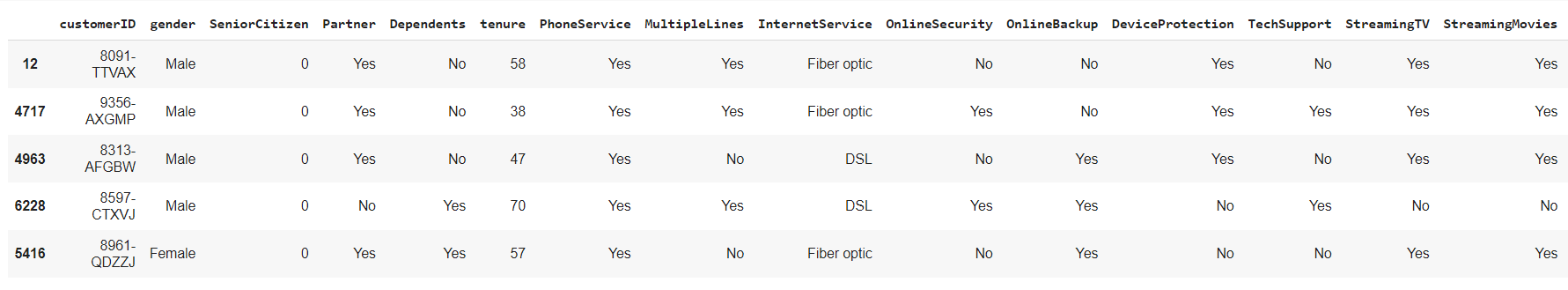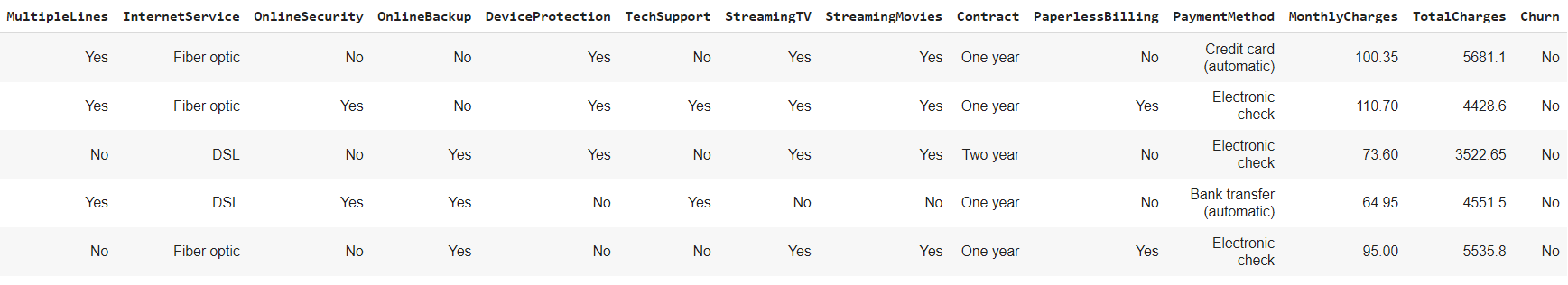Our focus is the ‘Churn’ column which contains the sample of subscriber churning or not.

Data Scrubbing
Next, we will check if the columns have any null values.

df.isnull().sum()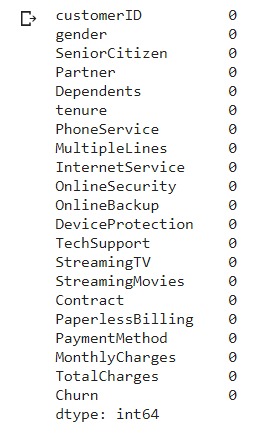The important thing is we do not have or minimal null values in the columns, so we can proceed with other cleaning.
Next we look data type of each value in the columns

df.info()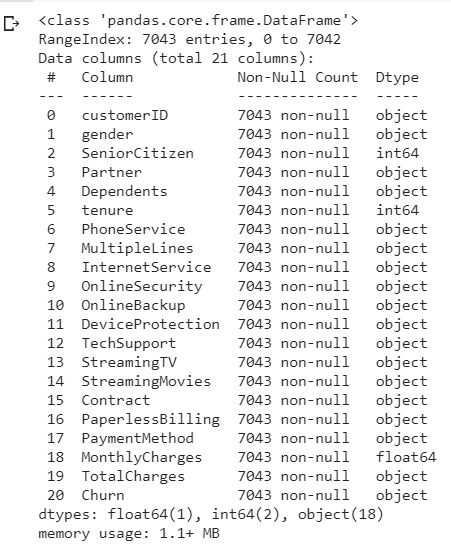Looking at each 20 column value data are object input except for 'SeniorCitizen', 'tenure' & 'MonthlyCharges' columns. For 'customerID' columns since that data type object is unique representing each subscriber, we do not need to clean this column and leave as it is. Accurate analyzing, require data to be in either integer or float which we will do.
Let check the rest of the 17 columns input data, and see if we can change it from object to numerical. We group the columns as col tor easier call up and use the function unique to check.

col = ['gender', 'SeniorCitizen', 'Partner', 'Dependents', 'PhoneService', 'MultipleLines', 'InternetService', 'OnlineSecurity', 'OnlineBackup', 'DeviceProtection', 'TechSupport', 'StreamingTV', 'StreamingMovies', 'Contract', 'PaperlessBilling', 'PaymentMethod', 'TotalCharges', 'Churn']
for column in col: print(column, ':', df[column].unique(), '\n'))

Let see the result.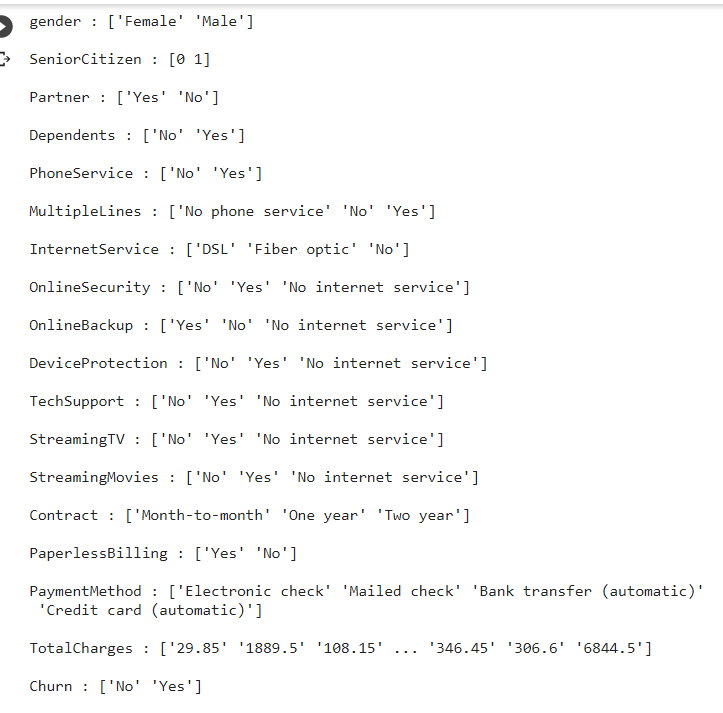There is a mixed input of data inside the table. We try to clean from left to right column and group it as much as we can for conversion/mapping in a simpler coding. First column 'gender'', we can convert Female, Male data object to numerical 0 & 1 respectively using mappping function as per below.

df['gender'] = df['gender'].map({"Female":0, "Male":1})

Next one is 'Partner' columns which have NO & Yes input, with 3 other columns as well. We group it under binary_columns and map it No, Yes to 0,1 respectively

binary_columns = ["Partner", "PhoneService", "Dependents", "PaperlessBilling", "Churn"] for column in binary_columns: df[column] = df[column].map({"No": 0, "Yes": 1})

Next column is 'MultipleLines'column has ['No phone service', 'No', 'Yes'] input. I think suffice to say we can group 'No phone service as No as well, so we map as [0,0,1] respectively.

df['MultipleLines'] = df['MultipleLines'].map({"No phone service":0, "No":0, "Yes":1, })

Moving on, is 'InternetService' columns which has ['DSL', 'Fiber optic', 'No'] input. From this, it is a bit akward to map it DSL or Fiber Optic as 0,1 where this input does not mean one is better than the other. To circumvent this, we use the dummy function by create additional columns for each input, and automatically assign 0,1 for them. Other columns 'Contract' and 'PaymentMethod', has the same unique input so we use the dummy function as well in one cell.

df = pd.get_dummies(data=df, columns=['InternetService'])
df = pd.get_dummies(data=df, columns=['Contract'])
df = pd.get_dummies(data=df, columns=['PaymentMethod'])

The next column to check is the 'OnlineSecurity' columns. It has 'No' ,'Yes' ,'No internet service'. Similar to 'MultipleLines'column, we can map 'No Internet Service' as No as in 0. We can also group with 5 other colums that has the same input.

binary2_columns = ['OnlineSecurity', 'OnlineBackup', 'DeviceProtection', 'TechSupport', 'StreamingTV', 'StreamingMovies']
for column in binary2_columns:
df[column] = df[column].map({"No": 0, "Yes": 1, "No internet service":0})

Lastly, the 'TotalCharges' column. From the input, it shows continous numbers as it should however it has being classify as object, probably wrongly formatted column. In order to rectify this, we can use the pandas.to_numeric method, with parameters "coerce" any errors in conversion, the value become NaN.

df['TotalCharges'] = pd.to_numeric(df['TotalCharges'], errors="coerce")

Now all columns has being converted to integer/float, we do a simple check by using the sample function.

df.sample(5)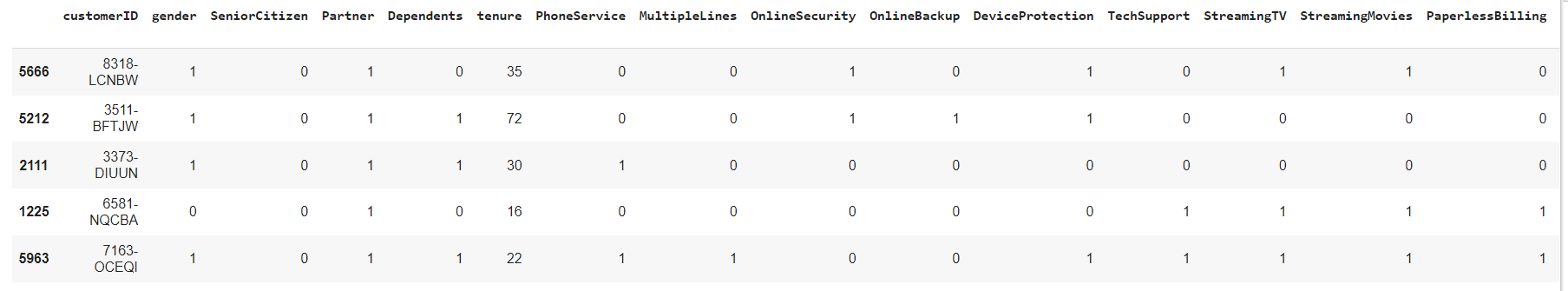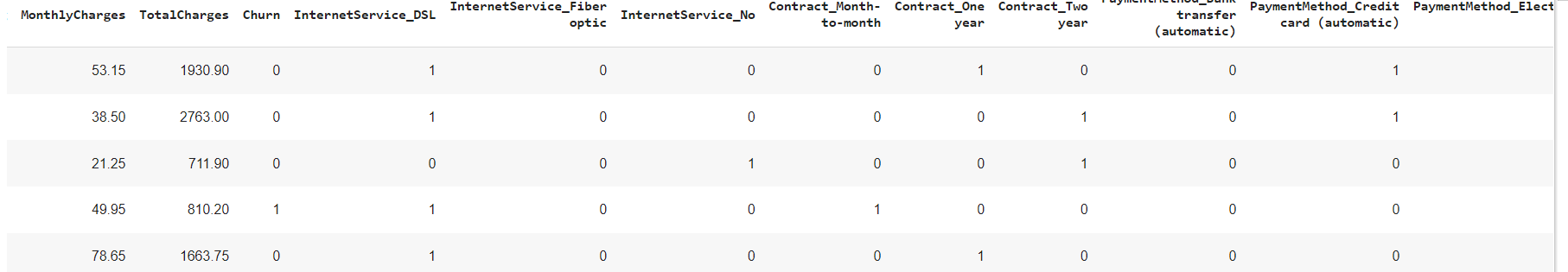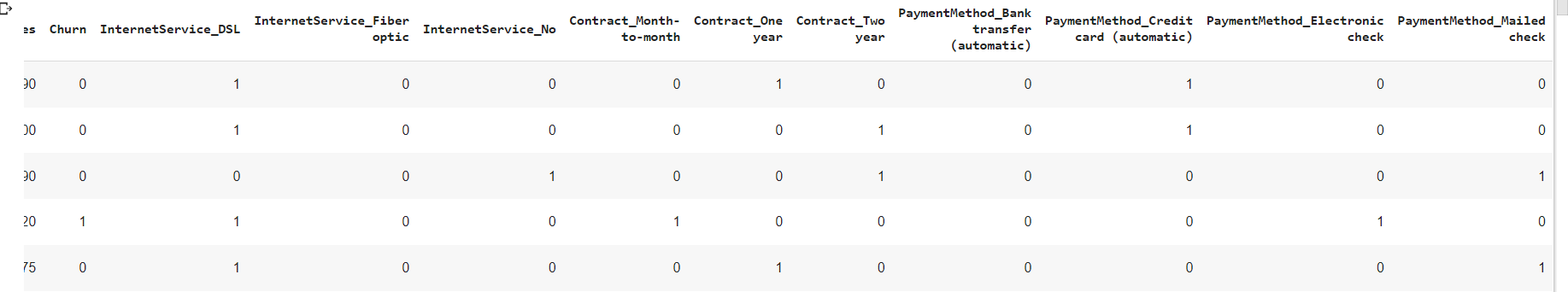We have a quick glance and see most columns has 0,1 input, and with addition a few columns as dummies as per our code before. We also recheck if there is any NaN values after our conversion column 'TotalCharges'

df.info()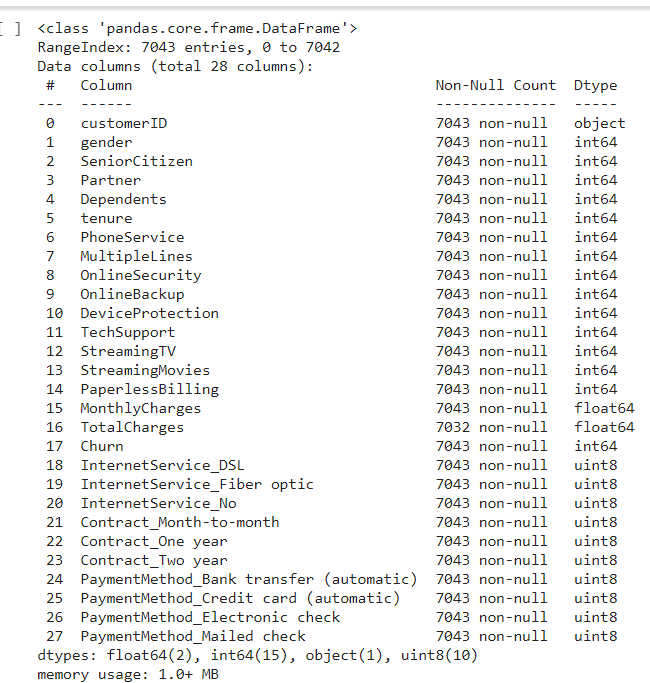All columns has being converted into integer and float for easy analyzing, now to check any missing data in the table. There is 11 missing input in the 'TotalCharges' column, by comparing 7,043 sample from other columns that has non null vales with 7,032 samples in 'Total Charges' column.
Since it is only 11 input from the total 7,043 count, i have decided to drop the missing row value from the column by using the function dropna since it is immaterial.

df = df.dropna()

Explore Data

For exploring the data after the data scrubing, i would like to use the descriptive analysis function on the data, so let's try that.

df.describe()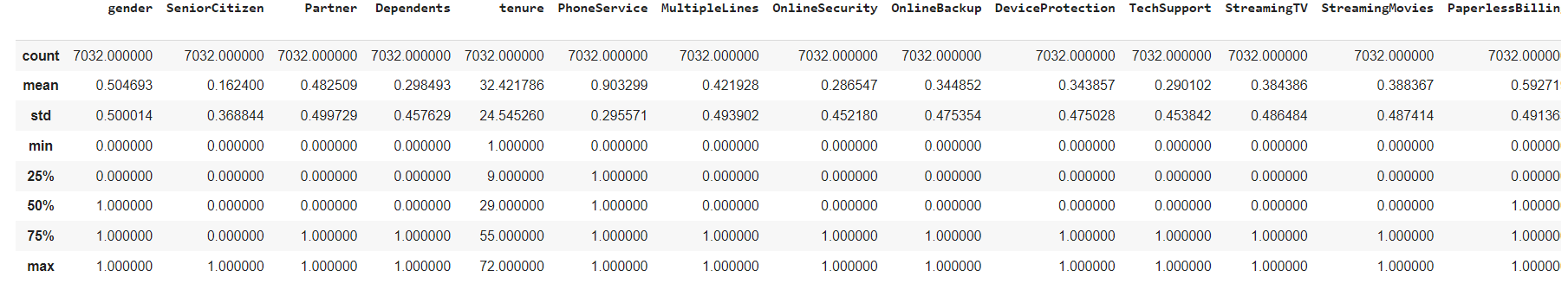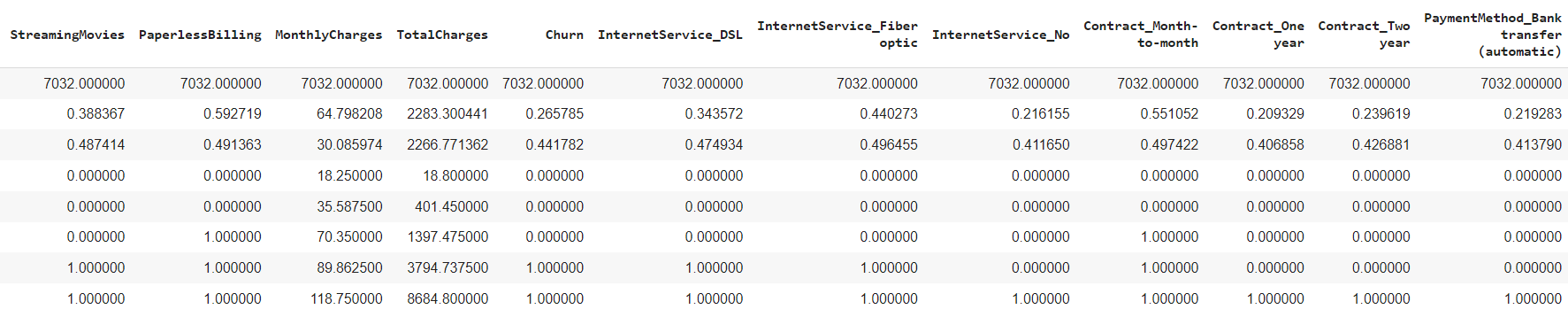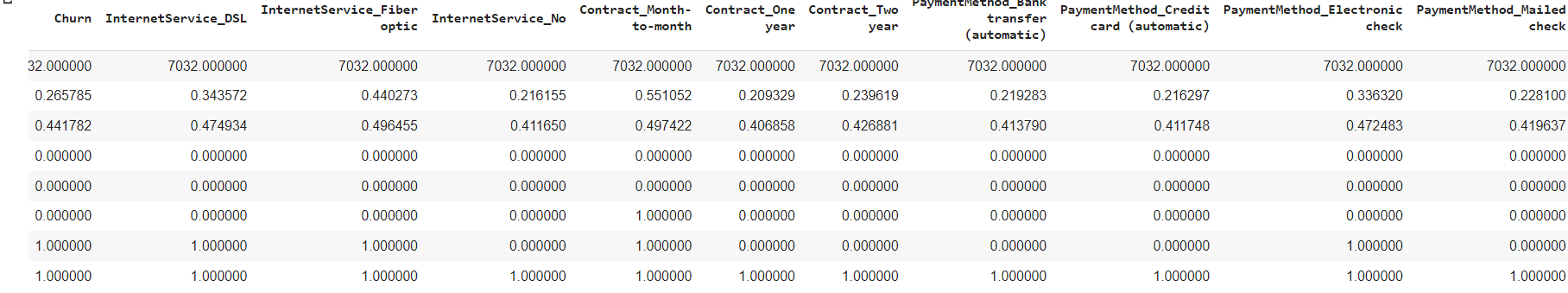We also can check that the all sample rows has being reduced to 7,032 input after dropna function
General summary on the descriptive analysis based on the describe function shown
1. The subscriber is divided around 50/50 by male & female
2. the standard deviation churn is low with 0.441 which means the mean has +- deviation of 0.441782 from the mean. The lower is better.
3. The Mean of 26.57% churn rate means that out of 7032 samples, around a quarter of total sample churned from subscribing from the telco line.
4. The average tenure for each customer out of 7032 samples is about 32 months (2 years and 8 months).

Another way to explore data is using test significant variables.Testing significant variables often is done with correlation.
The correlation can be find by using heatmap. By displaying a panda dataframe in Heatmap style, a visualization that is very useful in visualizing the concentration of values between two dimensions of a matrix. This helps in finding patterns and gives a perspective of depth.

Visualization Data as Heatmap
Lets try the heatmap function as per below.

sns.set(rc={'figure.figsize':(20,20)}) corr = df.corr() sns.heatmap(corr, annot=True,fmt=".2f")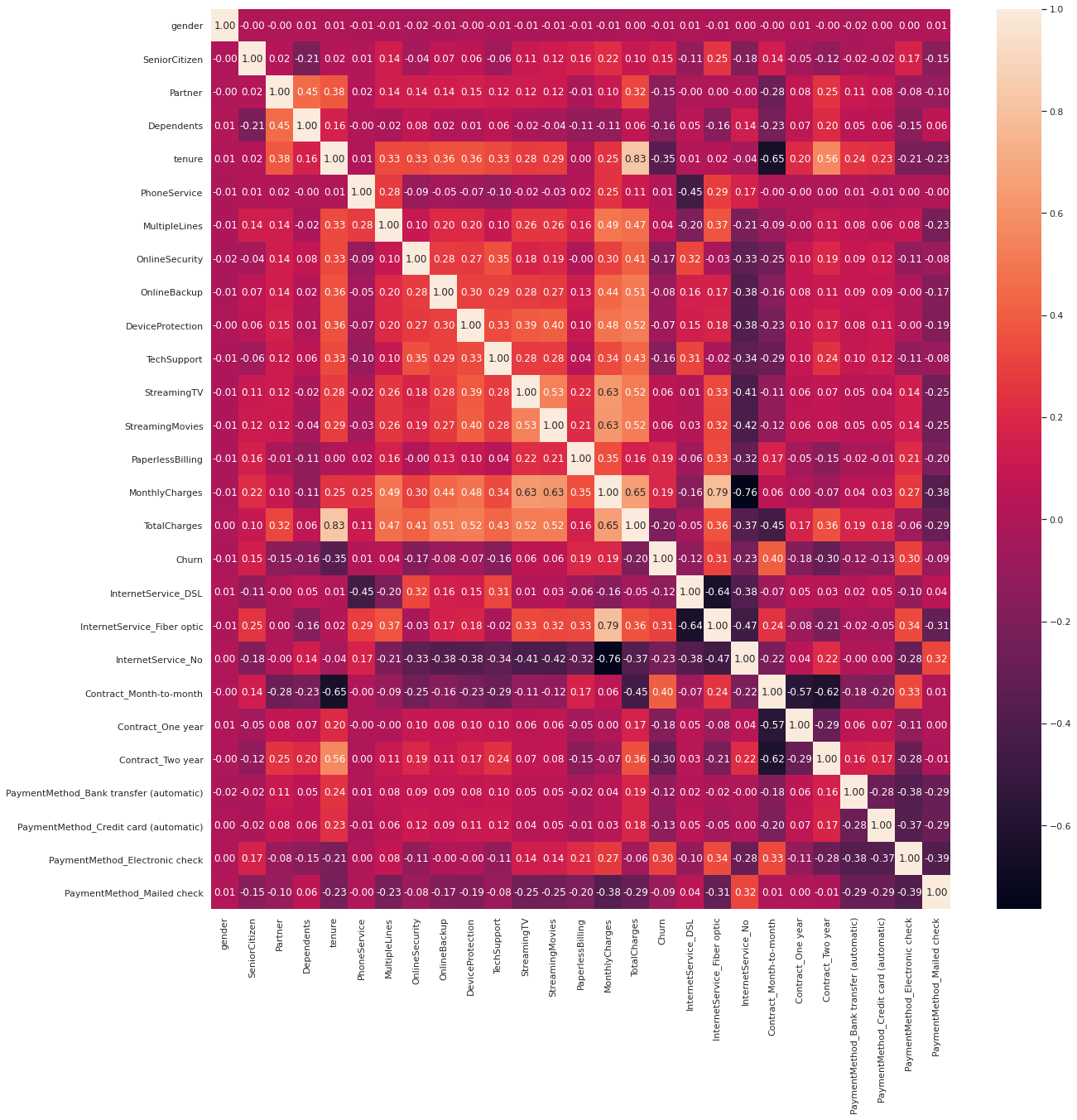From the heatmap, we can see a couple interesting correlation, the data churn and Contract_month_to month has a high correlation. This correlation could help us to find a batter model predictor for churn later on.
While we also could point out that churn has a compratively negative relationship with tenure as well
Lastly, we will utilise data visualisation to help us to identify significant patterns and trends in our data. We can gain a better picture through simple charts like line charts or bar charts to help us to understand the importance of the data.

Visualization Data as Countplot
Here we use Countplot, visualization that is very useful to show the counts of observations in each categorical bin using bars. Since we are focusing on Churn data, I will use all other columns as the x axis in the countplot to find which column has best relatioship with the churn with the code as per below.

main, subplots = plt.subplots(1, 4, figsize=(20,6))
sns.countplot(data=df, x="gender", hue="Churn", ax=subplots)
sns.countplot(data=df, x="SeniorCitizen", hue="Churn", ax=subplots)
sns.countplot(data=df, x="Partner", hue="Churn", ax=subplots)
sns.countplot(data=df, x="Dependents", hue="Churn", ax=subplots)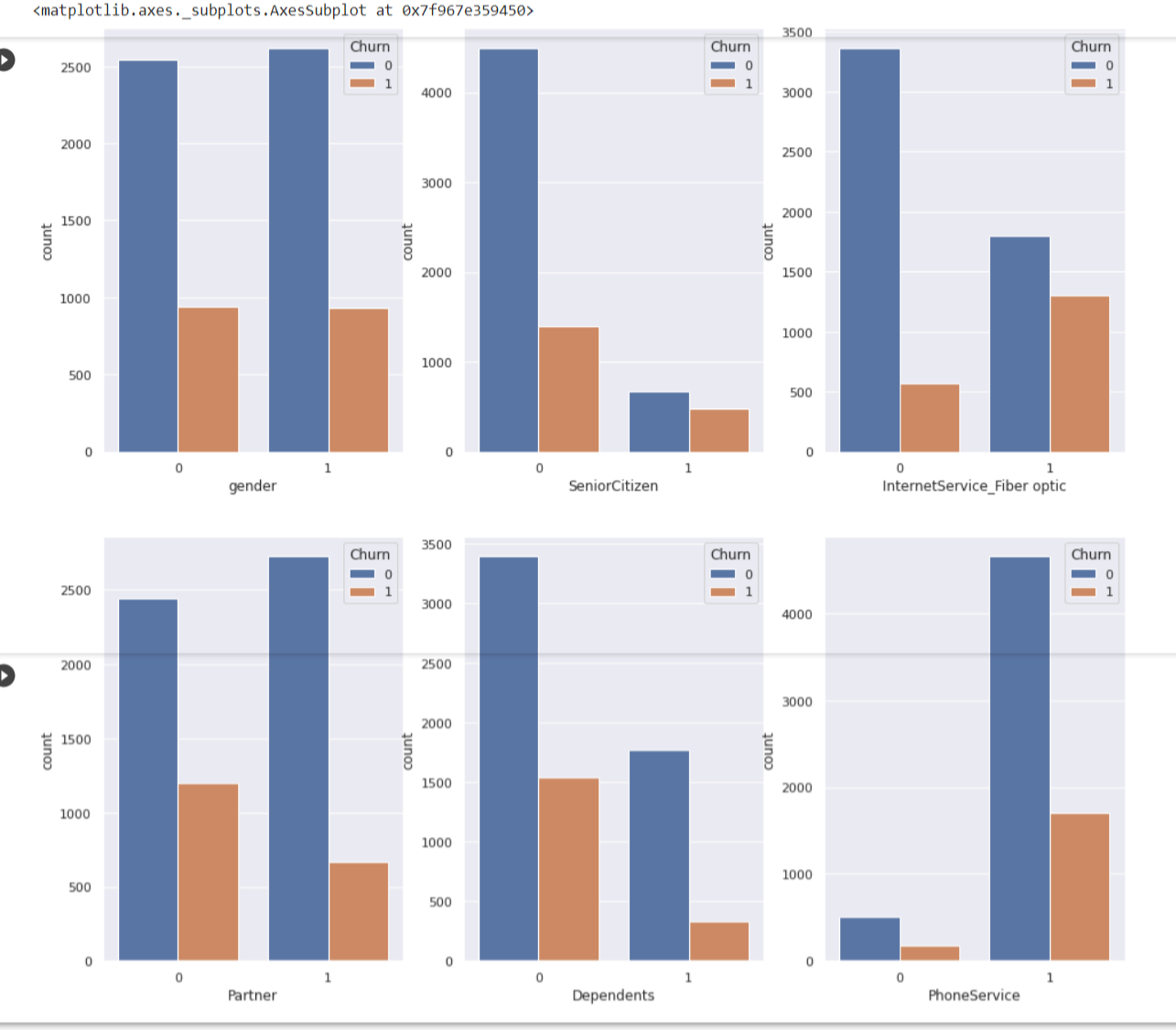Findings from countplots
1. Gender Female, Male has around the same count to churn
2. Senior Citizens, Partner and Dependents has the same trend where numbers of churning is quite low compare to non churning.
3. Subcscriber who has Internet Service Fiber Optic to churn are are not that different that much.
4. PhoneService has different trend altogether, where phone service and non phone service are already varies so much. Subscribers with phone service shows a large difference in not churning than churn

Visualization Data as Boxplot
Another visualization method, create boxplot, a visualization for graphically depicting groups of numerical data through their quartiles. We wil use data x= Churn data, I will use other columns as the y axis in the boxplot as per below

main, subplots = plt.subplots(1,3, figsize=(16,10))
sns.boxplot(data=df, x='Churn',y='tenure',ax=subplots)
sns.boxplot(data=df, x='Churn',y='MonthlyCharges',ax=subplots)
sns.boxplot(data=df, x='Churn',y='TotalCharges',ax=subplots)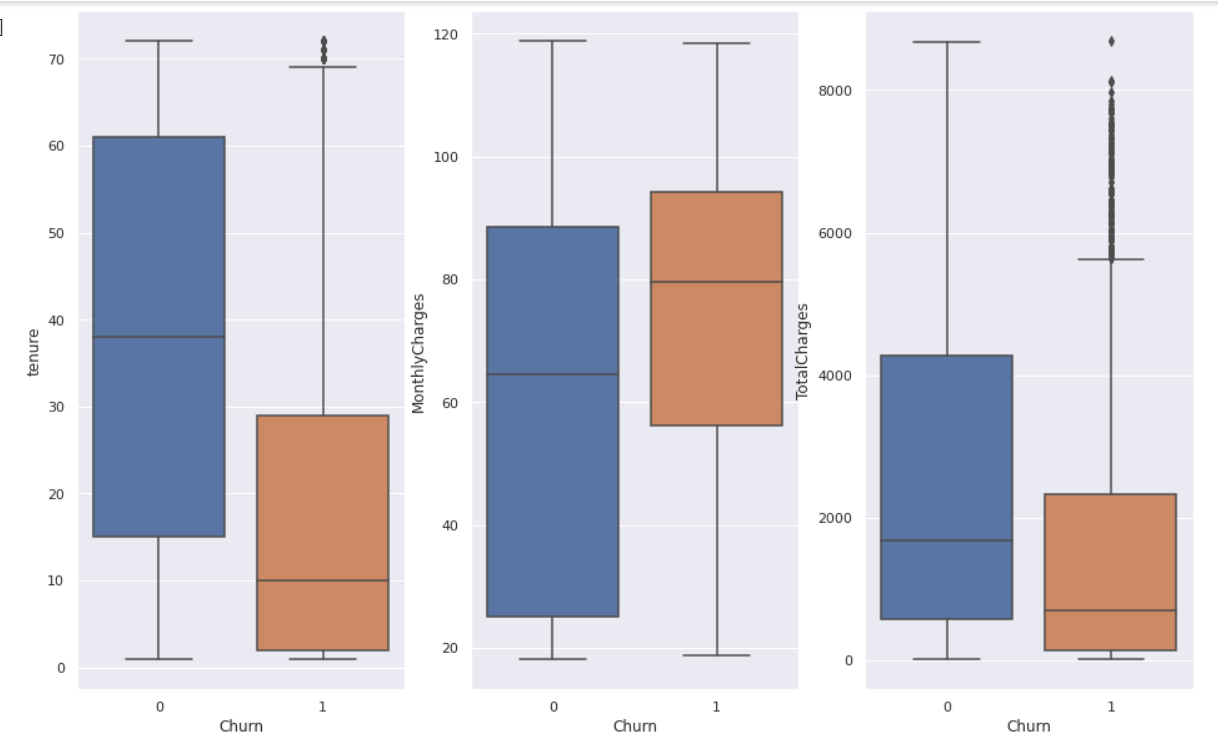The box extends from the Q1 to Q3 quartile values of the data, with a line at the median (Q2). The whiskers extend from the edges of box to show the range of the data.
Findinds from boxplot
1. You can find outliers in both tenure and Total Charges, they are data outside of Q3 as per the graph.
2. Both tenure and Total Charges to churn has also low 1st quartile percentage till the median
3. Monthly charges has a higher range in churn than the non churner.

Model Data
In modelling the data, not all your features or values are essential to predicting your model. What you need to do is to select the relevant ones that contribute to the prediction of results. For accuracy, we need to use as much data as we can but relevant as well. Here I will train models to forecasting future values.
As we work with datasets, a machine learning algorithm works in two stages. We usually split the data around 20%-80% between testing and training stages. We split a dataset into a training data and test data. For the columns i choose to include 'SeniorCitizen', 'Partner', 'PhoneService', 'DeviceProtection', 'OnlineSecurity', 'OnlineBackup', 'TechSupport', 'PaperlessBilling', 'tenure', 'MonthlyCharges', 'TotalCharges'.
Firstly, we group the columns involved as train_data, the features, data we used to predict the train, Churn as the train labels as it is what we wanted to train on.

columns = ['SeniorCitizen', 'Partner', 'PhoneService', 'DeviceProtection', 'OnlineSecurity', 'OnlineBackup', 'TechSupport', 'PaperlessBilling', 'tenure', 'MonthlyCharges', 'TotalCharges' ] train_data = df[columns] train_labels = df['Churn']

Importing Python Libraries
As usual, we need to import the specific pyton libraries which is sklearn train_test_split, decision tree and metrics as per below

from sklearn.model_selection import train_test_split
from sklearn import tree
from sklearn import metrics

Now we split arrays or matrices into random train and test subsets. The train size parametere is 30% proportion of the dataset to include in the train split. Another line is the decision tree model that we used to forecast decision, with parameter max_depth = 3, so it will at level 3 at max. And we called up the train data and train labels that was group before and use the function model.fit as Train Decision Tree Classifer

X_train, X_test, y_train, y_test = train_test_split(train_data, train_labels, test_size=0.3, random_state=1)
model = tree.DecisionTreeClassifier(max_depth = 3)
model.fit(train_data, train_labels)

And now we used the sklearn predictions in predict the response for test dataset. In addition we will we use metrics.accuracy_score calculate the model accuracy.

y_pred = model.predict(X_test)
print ("Accuracy:", metrics.accuracy_score(y_test, y_pred))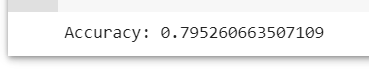Here after filtering and cleaning the data, we would get a 79.5% of accuracy if to model it in which i think that's a good indicator.

Visualizing Model Data as Decision Trees
Additionally, You can visualize the Decision Tree alogorithm using the sklearn graphiz library.

Importing Python Libraries
First we import libraries sklearn graphviz function for display and plot the tree

from sklearn.model_selection import train_test_split
from sklearn import tree
from sklearn import metrics

And we proceed with plotting where we call up back the columns

fcolumns = list(train_data.columns) dot_data = tree.export_graphviz(model, out_file=None, feature_names=columns, class_names=["No", "Yes"], filled= True, rounded = True) graph = graphviz.Source(dot_data) graph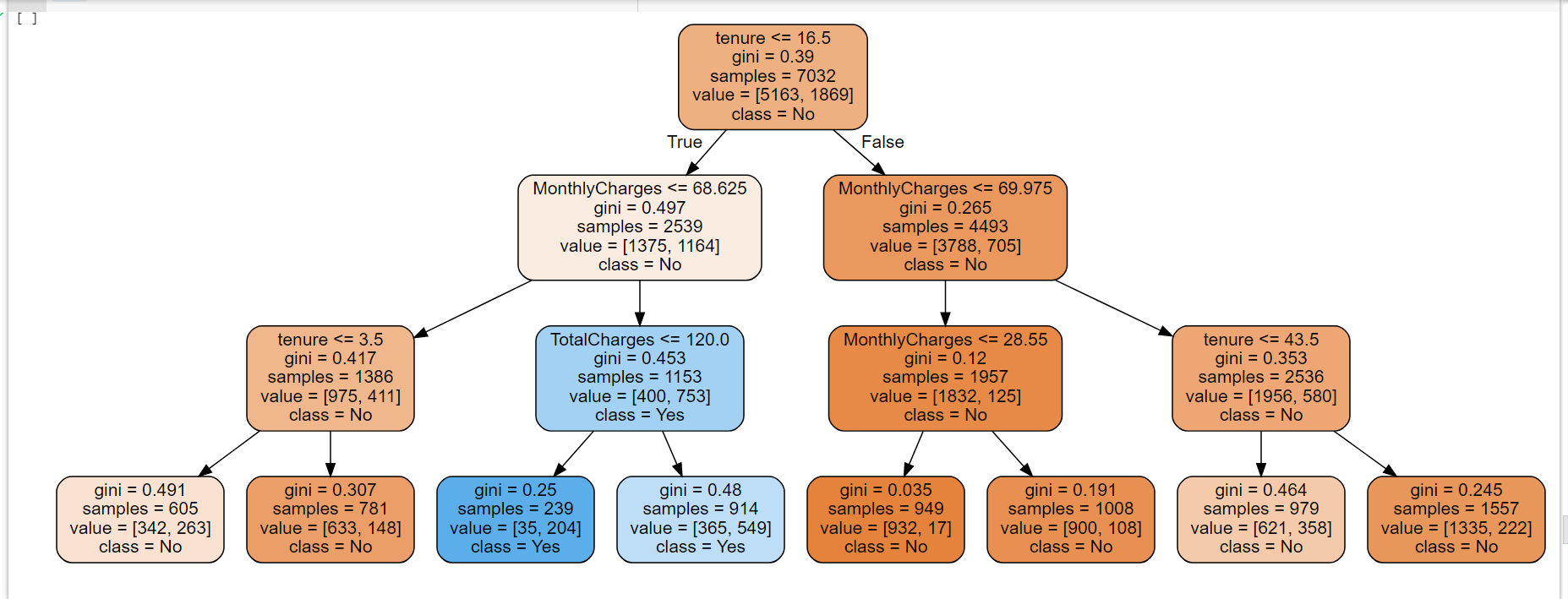Findinds from decison tree
1. Subscribers that has tenure that 16.5 months and monthly charges mroe than 68.25 are likely to churn with 35 out of the sample 7,032.
2. The probability increases of not churning if their tenure with the telco more than 15.5 months.

Using The Model
Lets recap the model with the colums involved are 'SeniorCitizen' 'Partner', 'PhoneService', 'DeviceProtection', 'OnlineSecurity', 'OnlineBackup', 'TechSupport', 'PaperlessBilling', 'tenure', 'MonthlyCharges', 'TotalCharges'.
Let's take a scenario where subscriber has phone service, Device protection,online backup,tech support, paperlessbilling, 12 months contract, Monthly Charges of RM80 and Total Charges of RM 1000, what is the prediction of churning?
"SeniorCitizen" :0,
"Partner": 0,
"PhoneService": 1,
"DeviceProtection": 1,
"OnlineSecurity": 0,
"OnlineBackup": 1,
"TechSupport":1,
"PaperlessBilling": 1,
"tenure": 12,
"MonthlyCharges": 80,
"TotalCharges":1000

Lets put scenario input inside model.prediction and see how it goes

model.predict([[0, 0, 1, 1, 0, 1, 1,1,12,80,1000]])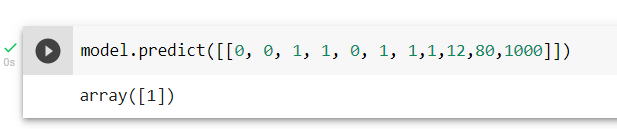Interpreting Data
The result 1 means customers with the scenario given would churn with accuracy model 79.5%.

Call To Actions/Suggestion to Telco Company To Retain Customers

1. Increase clients with longer Tenure - To attract them by roll out better packages locking in long term contracts.
2. Reduce Monthly Charges - To give some sort loyalty discounts or points to reduce monthly charges.
3. Reduce Total Charges - To set competitive charges in line with the market, like service charges, internet charges, overseas charges etc.

Other Factors

Other than relying on the model, we also need to consider external factor like current situation like this COVID, market competitiveness of Telco in the country and technology. One thing comes in mind is people are working from home & students are learning online, telcos need to readjust their packages to suit the current market need.

## Word CloudsTweets

# Making Word Clouds Based On Trump Tweets

The datasets and complete code can be found at the link below.

The method I am using to analyze the dataset is by using Data Science OSEMN framework.
In this portfolio, I am interested with words is he using the most in his tweets. How is he choosing these particular words to send his powerful messages, which effect instantly on stock market and the economic. Using visualization, creating a wordcloud with Donald Trump’s recent tweets to explore his most used words.

Importing Python Libraries
First, we need to import the necessary python libraries for this analysis. These include the basic packages such as pandas, numpy, os import path, PIL import inage, wordcloud as well stopwords,imagecolor generator and urllib and lastly matplotlib pyplot and inline.

import numpy as np
import pandas as pd
from os import path
from PIL import Image
from wordcloud import WordCloud, STOPWORDS, ImageColorGenerator
import urllib
import matplotlib.pyplot as plt
% matplotlib inline

Data Source
The data that I am using for this portfolio is the raw dataset in kaggle as per link prior. It is stated that the tweets are from July 2020 to October 2020 In a quick glance it contains columns and rows with mostly words & numbers in the table. I will use Python to perform all data analysis and visualization. This data is originally in a CSV file and we will convert it into a data frame by using Pandas and let’s take a look at what we have.

/main/realdonaldtrump.csv")
df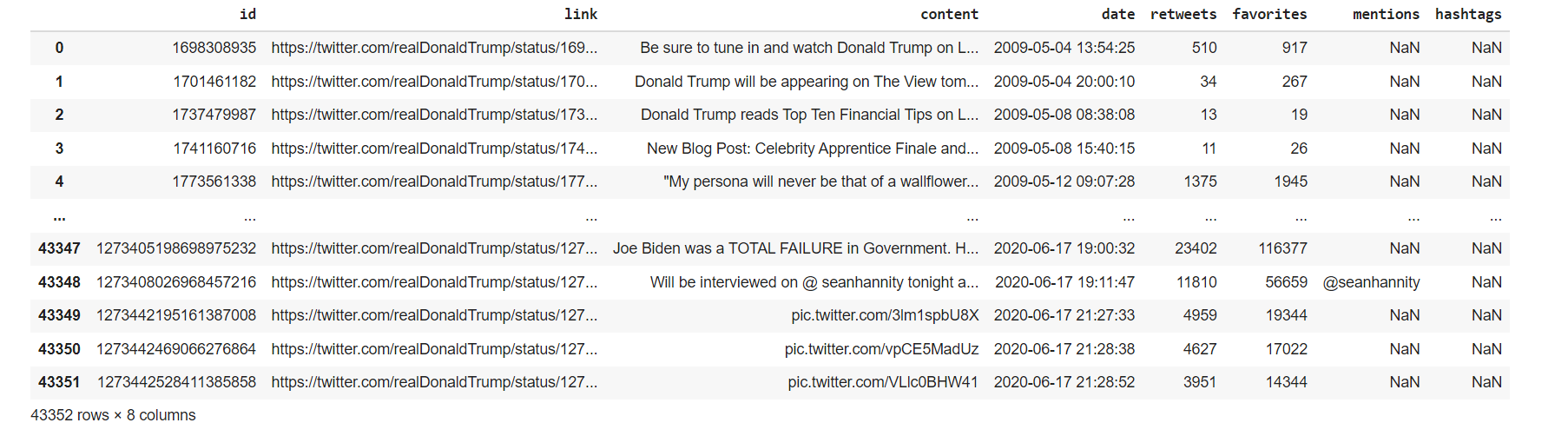Our focus is the ‘content’ column which contains the words of Trump tweet. Mind on the NaN values as well.

Data Scrubbing
Next, we will check if the columns have any null values.

df.isnull().sum()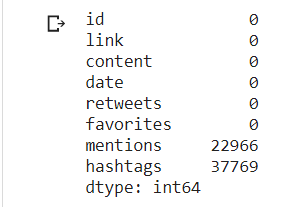There are NaN values in 'mentions'and 'hashtags'column but our focus is the ‘content’ column which contains the tweets. We can drop the other columns but I will just keep them in the data frame since they won’t impact my wordcloud.

Next we look data type of each value in the columns focusing on the 'content' column.

df.dtypes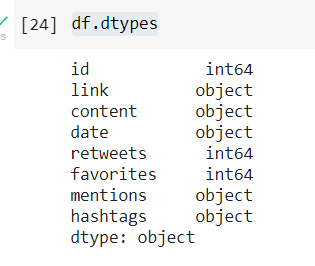stopwords = set(STOPWORDS)Visualizing Data as Wordcloud
One of the function that we want use as one of the parameter is stopwords where it will ignore commonly used words such “the”, “a”, “an”, “in” Set it and use another line code with the plotting pyplot,Imagegenerator, and other parameters like width and background color

stopwords = set(STOPWORDS)

def wordcloud_generator(data, title=None):
wordcloud = WordCloud(width = 1000, height = 1000,
background_color ='white',
stopwords=stopwords,
min_font_size = 10
).generate(" ".join(data.values))

# plot the WordCloud image
plt.figure(figsize = (16, 20), facecolor = None)
plt.imshow(wordcloud, interpolation='bilinear')
plt.axis("off")
plt.title(title,fontsize=30)
plt.show()Now we use wordcloud function and put a title on the image "Most used words in Trump Tweets"

wordcloud_generator(df['content'], title="Most used words in Trump Tweets")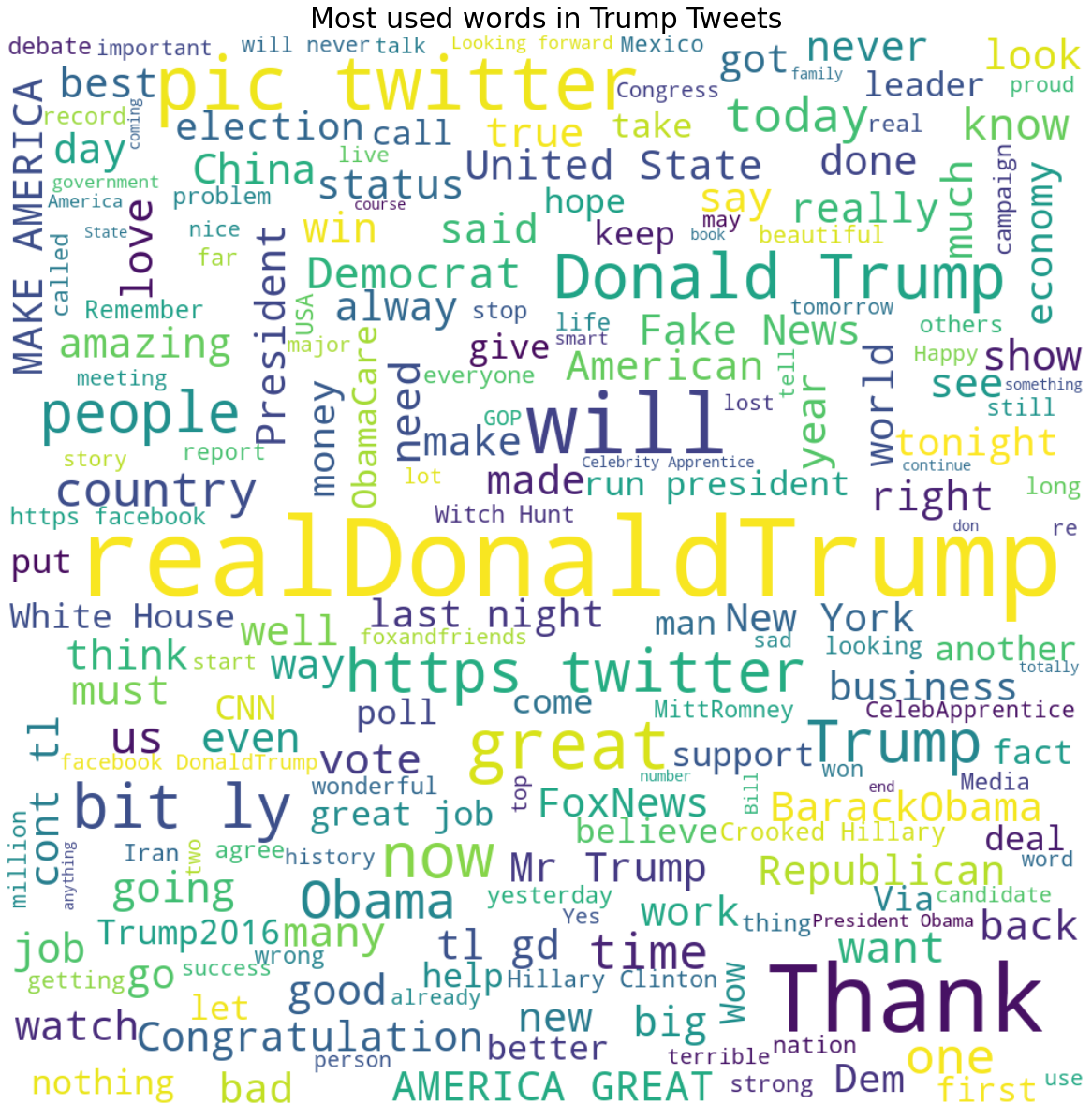Here, we could see trump words in different sizes, is organized as per picture above. For a better visualization, I want to use a mask image Trump and the words are organized accordingly to the mask body. Mask Images can be foound in google image, preferably a PNG as it will be nicer. Using the imported libraries urlib and numpy, we can callup the image mask as per line below

And then for our reference we plot the mask image according to our needed width and sizes as the line below using plt function to check it.

fig = plt.figure(figsize=(16, 20))

plt.axis('off')
plt.show()Looks like the image mask is ok, here we proceed to called again the line to visualize the word cloud but with a new parameter trump_mask

def wordcloud_generator(data, title=None):
wordcloud = WordCloud(width = 1000, height = 1000,
background_color ='white',
stopwords=stopwords,
min_font_size = 10
).generate(" ".join(data.values))

# plot the WordCloud image
plt.figure(figsize = (16, 20), facecolor = None)
plt.imshow(wordcloud, interpolation='bilinear')
plt.axis("off")
plt.title(title,fontsize=30)
plt.show()

wordcloud_generator(df['content'], title="Most used words in Trump Tweets")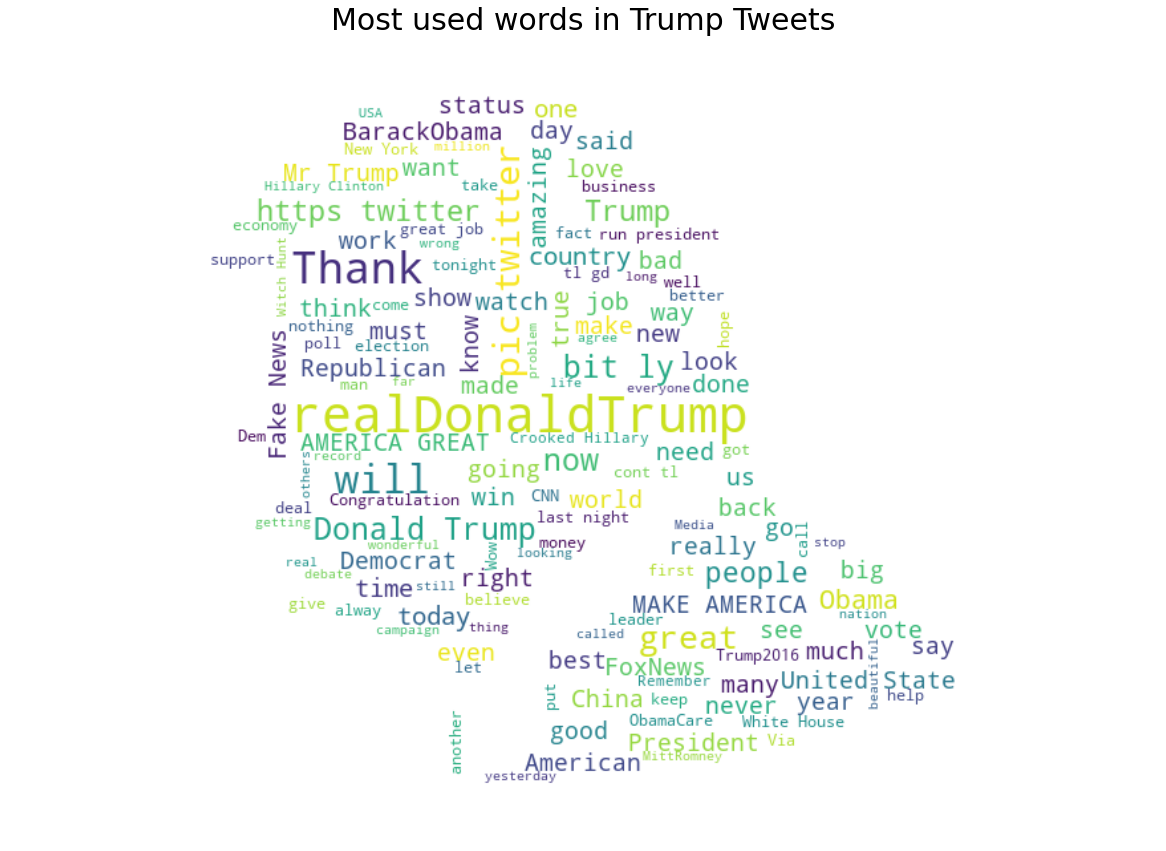Alright, now we have word cloud of Trump Tweet in his own Trump mask image with his iconic and unique hairstyle.

Interpreting Data
Whoever been keeping tabs on Donald’s twitter, will probably be familiarised with his tweets. Most tweeted words contain “Biden”, “America Great” and “Fake News”. It is also interesting that even after he won presidential election he still keep mentioning “BarackObama” which is the most tweeted words.
Conclusion
Wordclouds are useful for data exploration and analysis in NLP projects. They are great to visualize words in a creative way. This visualization can add values to other projects as well.